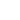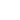+86 571 56668956
Language:
Home / News / Others /

Permanent magnet synchronous motor

Issue Time:2020-04-17

Definition of motor

As the name implies, motor is a kind of power component that converts electrical energy and mechanical energy. When electrical energy is converted into mechanical energy, the motor shows the operating characteristics of the motor; when electrical energy is converted into mechanical energy, the motor shows the operating characteristics of the generator. The motor is mainly composed of rotor, stator winding, speed sensor, housing, cooling and other components.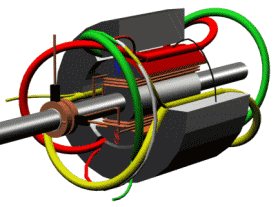Classification of motor

Divided by structure and working principle: DC motor, asynchronous motor, synchronous motor.

Divided by the type of working power: it can be divided into DC motor and AC motor.

Permanent magnet synchronous motor

Permanent refers to adding permanent magnets when manufacturing the rotor of the motor, so that the performance of the motor is further improved. The so-called synchronization means that the rotation speed of the rotor is always consistent with the current frequency of the stator winding. Therefore, by controlling the frequency of the input current of the stator winding of the motor, the speed of the electric vehicle will be finally controlled. How to adjust the current frequency is the problem to be solved by the electronic control part.

Principle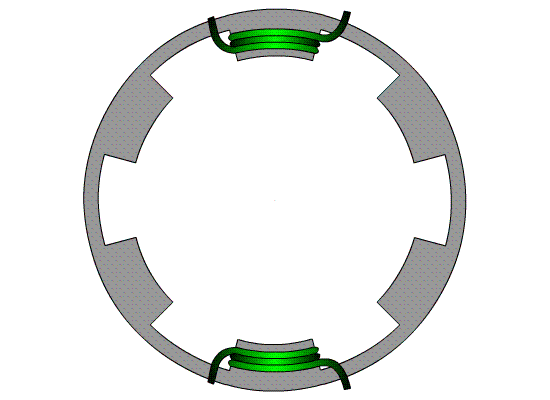A set of coils is energized

First of all, we assume that a group of coils (green group) is energized. After energization, it can be regarded as a vertical coil energization, then it will generate a magnetic field similar to a bar magnet (of course, the middle is empty, the magnetic field lines are expanded instead Tightly packed like a bar magnet). Therefore, when the coil is energized, it is equivalent to putting a bar magnet on the stator.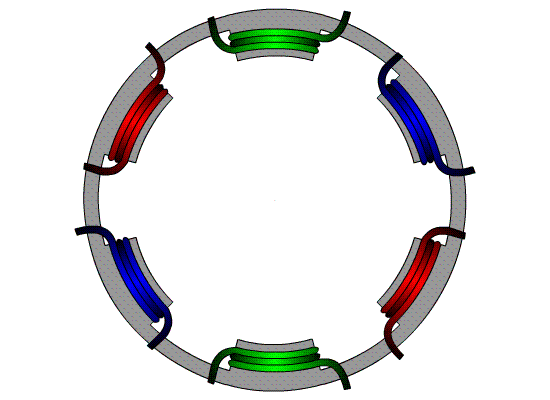Three sets of coils are energized

On the left, only one set of coils is energized. The magnetic field of the three sets of coils is equivalent to three bar magnets placed in the shape of "meter" inside the stator. When the three sets of coils are energized in sequence, a continuously rotating magnetic field is generated.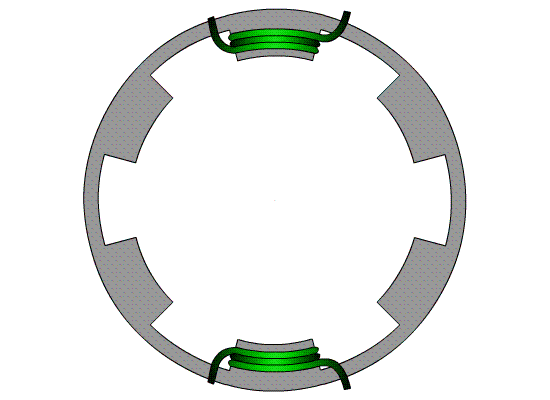Bar magnet is rotated

Put a bar magnet in the constantly rotating magnetic field, then the bar holding iron will rotate with the rotating magnetic field due to the magnetic moment. Since the magnetic force is instantaneous, the magnet can be immediately stressed when the coil changes. That's the Synchronize

Ok, now the three sets of coils are energized in turn to form a rotating magnetic field, but this magnetic field is not smooth. Because the magnetic force of the coil will change during the switching on. At the same time, if the stator has only one magnet, the force it receives is very small, and because the magnetic poles are unevenly distributed (only in two directions of the diagonal), the force is also an uneven wave line. Therefore, in order to solve this problem, more magnets (here, 4) are placed on the stator to form a stator magnetic group, thus forming a permanent magnet rotor with SN pole spacing. At this time, the three sets of coils are constantly changing the direction of energization. The stator generates three changing magnetic fields, and the rotor in the magnetic field has a magnet in any direction, so it rotates steadily.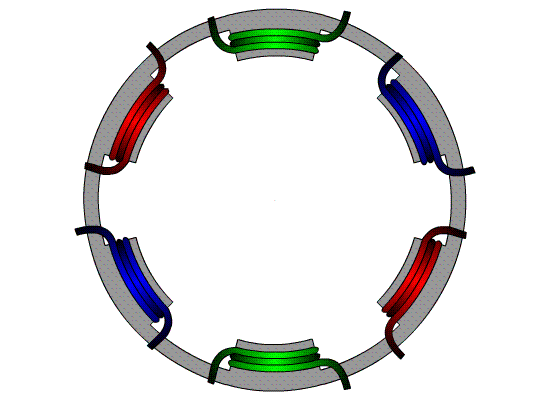Characteristics of permanent magnet synchronous motor

Permanent magnet motors have a higher power / mass ratio, smaller size, lighter weight, greater output torque than other types of motors, and the motor's limit speed and braking performance are also excellent. Therefore, permanent magnet synchronous motors have become The electric motor most used in electric vehicles today. However, when the permanent magnet material is subjected to vibration, high temperature and overload current, its magnetic permeability may decrease, or demagnetization may occur, which may reduce the performance of the permanent magnet motor. In addition, rare-earth permanent magnet synchronous motors use rare-earth materials, and the manufacturing cost is not stable